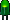• Home• Patreon• Links• Books
Number Converting:

# Number Systems

There are infinite ways to represent a number. The four commonly associated with modern computers and digital electronics are: decimal, binary, octal, and hexadecimal.

Decimal (base 10) is the way most human beings represent numbers. Decimal is sometimes abbreviated as dec.

Decimal counting goes:
0, 1, 2, 3, 4, 5, 6, 7, 8, 9, 10, 11, 12, 13, 14, 15, 16, 17, 18, and so on.

Binary (base 2) is the natural way most digital circuits represent and manipulate numbers. (Common misspellings are “bianary”, “bienary”, or “binery”.) Binary numbers are sometimes represented by preceding the value with '0b', as in 0b1011. Binary is sometimes abbreviated as bin.

Binary counting goes:
0, 1, 10, 11, 100, 101, 110, 111, 1000, 1001, 1010, 1011, 1100, 1101, 1110, 1111, 10000, 10001, and so on.

Octal (base 8) was previously a popular choice for representing digital circuit numbers in a form that is more compact than binary. Octal is sometimes abbreviated as oct.

Octal counting goes:
0, 1, 2, 3, 4, 5, 6, 7, 10, 11, 12, 13, 14, 15, 16, 17, 20, 21, and so on.

Hexadecimal (base 16) is currently the most popular choice for representing digital circuit numbers in a form that is more compact than binary. (Common misspellings are “hexdecimal”, “hexidecimal”, “hexedecimal”, or “hexodecimal”.) Hexadecimal numbers are sometimes represented by preceding the value with '0x', as in 0x1B84. Hexadecimal is sometimes abbreviated as hex.

0, 1, 2, 3, 4, 5, 6, 7, 8, 9, A, B, C, D, E, F, 10, 11, and so on.

All four number systems are equally capable of representing any number. Furthermore, a number can be perfectly converted between the various number systems without any loss of numeric value.

At first blush, it seems like using any number system other than human-centric decimal is complicated and unnecessary. However, since the job of electrical and software engineers is to work with digital circuits, engineers require number systems that can best transfer information between the human world and the digital circuit world.

It turns out that the way in which a number is represented can make it easier for the engineer to perceive the meaning of the number as it applies to a digital circuit. In other words, the appropriate number system can actually make things less complicated.

## Fundamental Information Element of Digital Circuits

Almost all modern digital circuits are based on two-state switches. The switches are either on or off. It doesn’t matter if the switches are actually physical switches, vacuum tubes, relays, or transistors. And, it doesn’t matter if the 'on' state is represented by 1.8 V on a cutting-edge CPU core, -12 V on a RS-232 interface chip, or 5 V on a classic TTL logic chip.

Because the fundamental information element of digital circuits has two states, it is most naturally represented by a number system where each individual digit has two states: binary.

For example, switches that are 'on' are represented by '1' and switches that are 'off' are represented by '0'. It is easy to instantly comprehend the values of 8 switches represented in binary as 10001101. It is also easy to build a circuit to display each switch state in binary, by having an LED (lit or unlit) for each binary digit.

## Making Values More Compact

“Binary digit” is a little unwieldy to say over and over, so the term was contracted to “bit”. Not only is the term “binary digit” a little unwieldy, but so is the length of a binary number, since each digit can only represent one switch. As digital circuits grew more complex, a more compact form of representing circuit information became necessary.

An octal number (base 8) can be up to 1/3 the length of a binary number (base 2). 8 is a whole power of 2 (23=8). That means three binary digits convert neatly into one octal digit.

A hexadecimal number (base 16) can be up to 1/4 the length of a binary number. 16 is a whole power of 2 (24=16). That means four binary digits convert neatly into one hexadecimal digit.

Unfortunately, decimal (base 10) is not a whole power of 2. So, it is not possible to simply chunk groups of binary digits to convert the raw state of a digital circuit into the human-centric format.

Let’s see how to convert a number from binary...